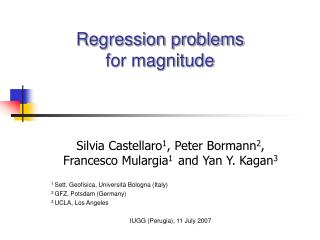DownloadDownload PresentationRegression problems for magnitude

# Regression problems for magnitude

Download Presentation## Regression problems for magnitude

- - - - - - - - - - - - - - - - - - - - - - - - - - - E N D - - - - - - - - - - - - - - - - - - - - - - - - - - -
##### Presentation Transcript

1. Regression problems for magnitude Silvia Castellaro1, Peter Bormann2, Francesco Mulargia1 and Yan Y. Kagan3 1 Sett. Geofisica, Università Bologna (Italy) 2 GFZ, Potsdam (Germany) 3 UCLA, Los Angeles IUGG (Perugia), 11 July 2007

2. The need for a unified magnitude • A large variety of earthquake size indicator exists (ms, mb, md, mL, M0, Me, Mw ,etc.) • Each one with a different meaning

3. Ignoring the fact that a single indicator of size may be inadequate in seismic hazard estimates, • The state of the art is to use on account of its better definition in seismological terms Mw

4. The magnitude conversion problem In converting magnitude, • It is commonly assumed that the relation Mx – My is linear (this is justified as long as none of them shows a much stronger saturation than the other) • Least-Squares Linear Regression is so popular that it is mostly applied without checking whether its basic requirements are satisfied

5. Linear Least-Squares RegressionBASIC ASSUMPTIONS • The uncertainty in the independent variable is at least one order of magnitude smaller than the one on the dependent variable, • Both data and uncertainties are normally distributed, • Residuals are constant.

6. Fail to satisfy the basic assumptions may: • Lead to wrong magnitude conversions, • Have severe consequences on the b-value of the Gutenberg-Richter magnitude-frequency distribution, which is the basis for probabilistic seismic hazard estimates

7. Which regression relation?

8. Here we focus on the performance of • = s2y /s2x

9. SR Standard least-squares Regression ISR Inverse Standard least-squares Regression GOR General Orthogonal Regression OR Orthogonal Regression. Special case of GOR with s2x~ s2y h =1 s2x 0, s2y > 0 Y  b X + a s2x> 0, s2y  0 Y  b X + a s2x> 0, s2y > 0 Y = b X + a

10. On normally, log-normally and exponentially distributed variables On normally, log-normally and exponentially dstributed errors On different amount of errors It has already been demonstrated that GOR produces better results than SR/ISR (Castellaro et al., GJI, 2006)

11. GOR SR ISR Example: (X, Y) exponentially distributed, Exponentially distributed errors added to X and Y, True slope (b) = 1.

12. However the point with GOR is • That the error ratio between the y and the x variables (h = s2y /s2x) needs to be known. • In practice h is mostly ignored since the seismological data centers do not publish standard deviations for their average event magnitudes.

13. To define the performance of the different procedures we run enough simulations to cover the ranges of • Slopes b • Ratios h between variances • Absolute values of errors sx,sy which may be enocuntered when converting magnitudes

14. Parameters used in the simulations In order to produce realistic simulations, parameters are inferred from the study of CENC (Chinese Earthquake Network Center), GRSN (German Regional Seismic Network) and Italian official catalogues • 0.5 < btrue < 2 • 0.05 < sx, sy < 0.50 • 0.25 <h < 0.3

15. Generation of the datasets 1) 103 couples of magnitudes (Mx, My) with 3.5 < Mx, My < 9.5 2) Sampled from exponential distribution (Utsu, 1999, Kagan, 2002, 2002b, 2005, Zailiapin et al. 2005) 3) From (Mx, My) to (mx, my) by addition of errors sampled from Gaussian distributions with deviations sx and sy • Steps 1) to 3) are repeated 103 times and 103 SR, ISR, GOR and OR regressions are performed to obtain the average bSR, bISR, bGOR, bOR and their deviations.

16. Results • GOR is always the best fitting procedure However, if h is unknown…

17. Attention should be paid to the mb-MS relation which is not linear (due to saturation of the short-period mb for strong earthquakes), has an error ratio of about 2 and usually a rather large absolute scatter in the mb data

18. If nothing is known about the variable variances, compare your case to the whole set of figures posted in www.terraemoti.net to get some examples for chosing the best regression procedure

19. A typical Italian dataset Italian earthquakes in 1981-1996. s computed for each earthquake each time it was recorded by at least 3 stations.

20. Mw-ms

21. Mw-mL

22. Mw-mb

23. The magnitude conversion problem may appear a solved problem while it is not! • For example, some authors in the BSSA (2007) state “this work likely represent the final stage of calculating local magnitude relation ML-Md by regression analysis…” but they forgot to consider the variable errors at all! It follows that…

24. BSSA, (2007) While the most realistic result should be…

25. The use of SR without any discussion on the applicability of the model is unfortunately still too common

26. The problem of the magnitude conversion can of course be approached also through other techniques IN EUROPE • Panza et al., 2003; Cavallini and Rebez, 1996; Kaverina et al., 1996; Gutdeutsch et al., 2002; Grünthal and Wahlström, 2003; Stromeyer et al., 2004: • Apply the OR for magnitude-intensity relations • but h = 1 • Gutdeutsch et al., 2002 finds the mL-ms relation through OR (h = 1) for the Kàrnik (1996) catalouge of central-southern Europe • Grünthal and Wahlström, 2003 applied the c2 regression to central Europe • Gutdeutsch and Keiser are studing the c2 regressions for magnitudes

27. The software to run SR, ISR, OR and GOR is available on www.terraemoti.net